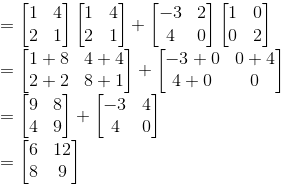# Selina Solutions Concise Maths Class 10 Chapter 9 Matrices Exercise 9(C)

This exercise primarily deals with the multiplication of matrices. Students who wish to secure good marks in their exams can make use of the Selina Solutions for Class 10 Maths. The solutions are created by experts in the subject having vast academic experience. The solutions of the Concise Selina for Class 10 Maths Chapter 9 Matrices Exercise 9(C) are available in PDF format enclosed in the links given below.

## Selina Solutions Concise Maths Class 10 Chapter 9 Matrices Exercise 9(C) Download PDF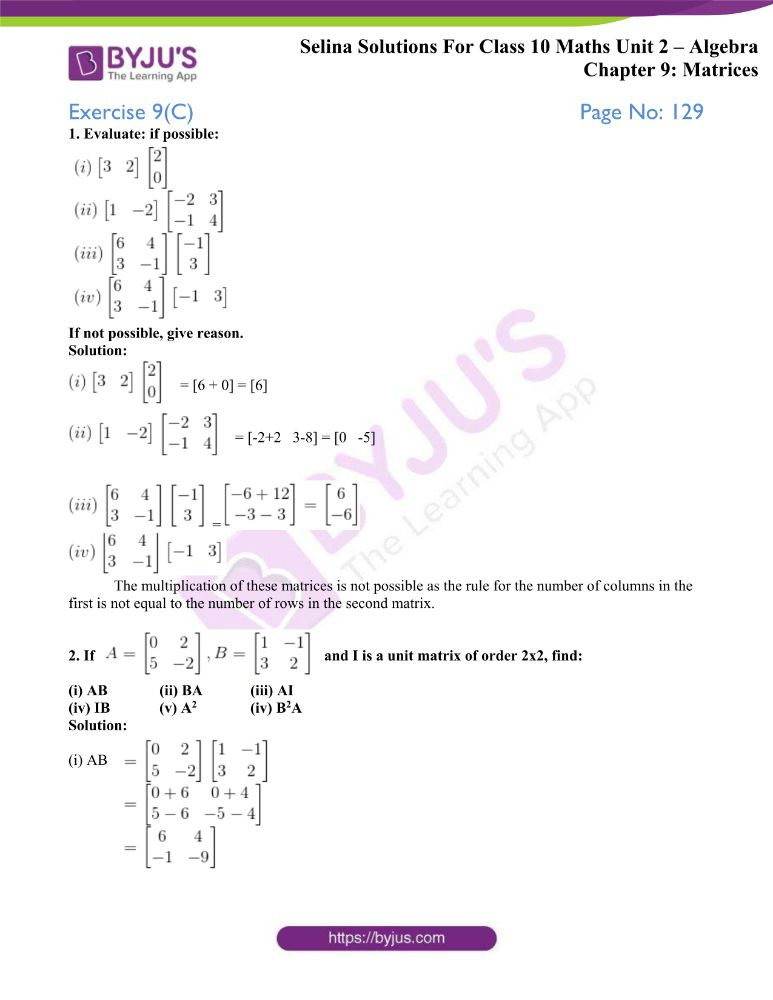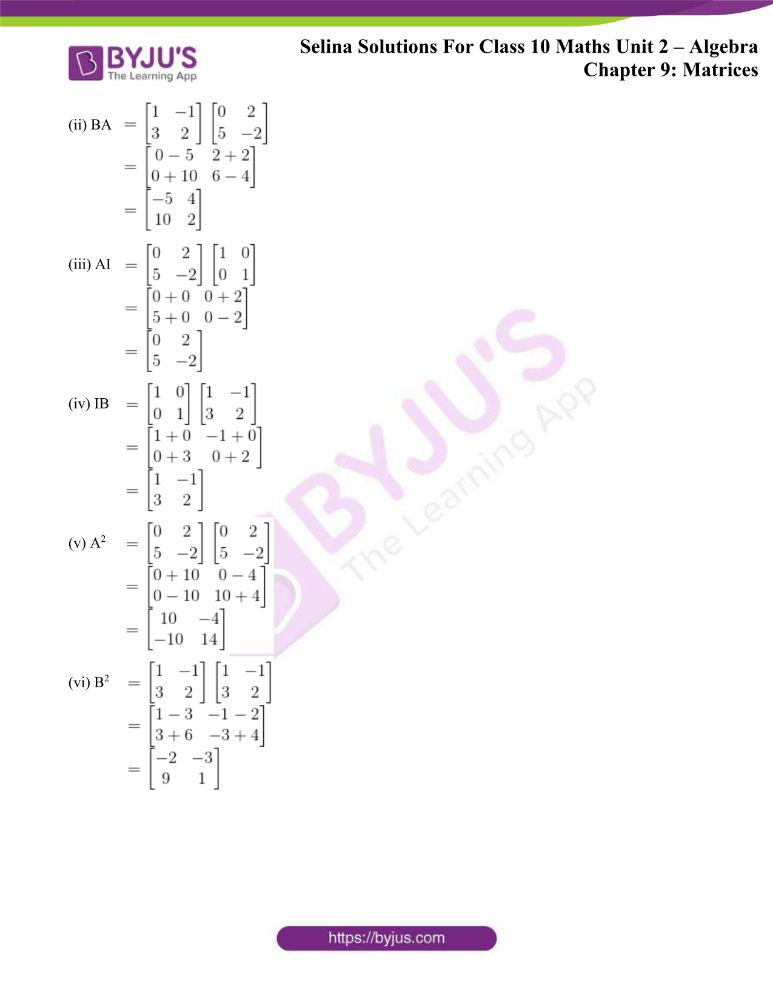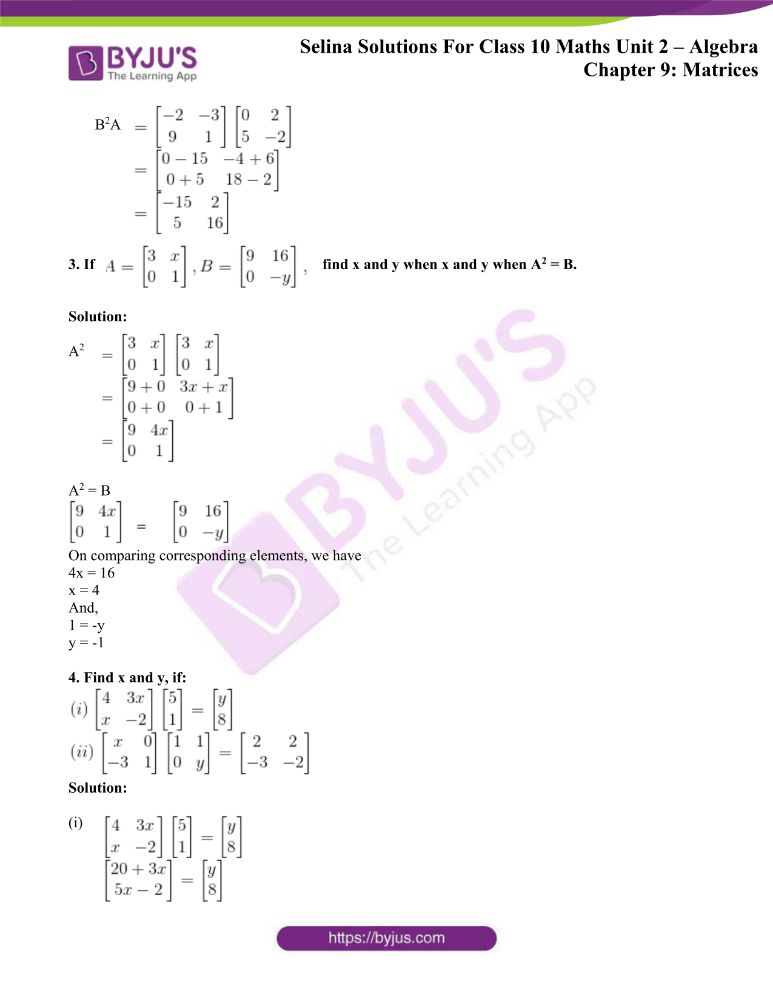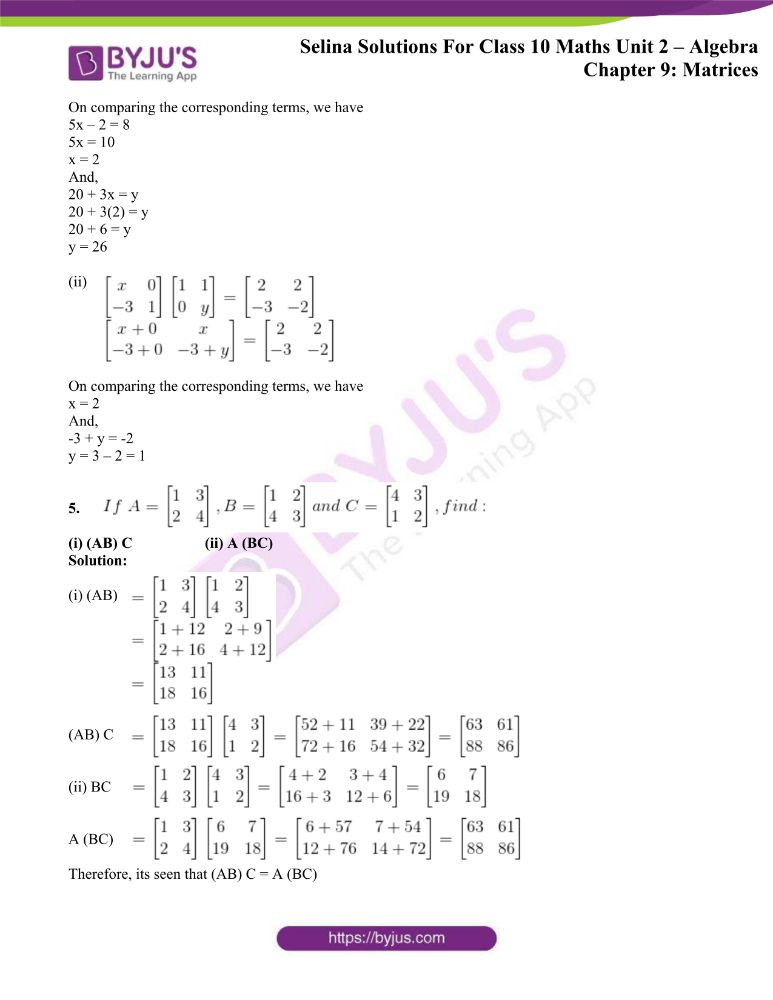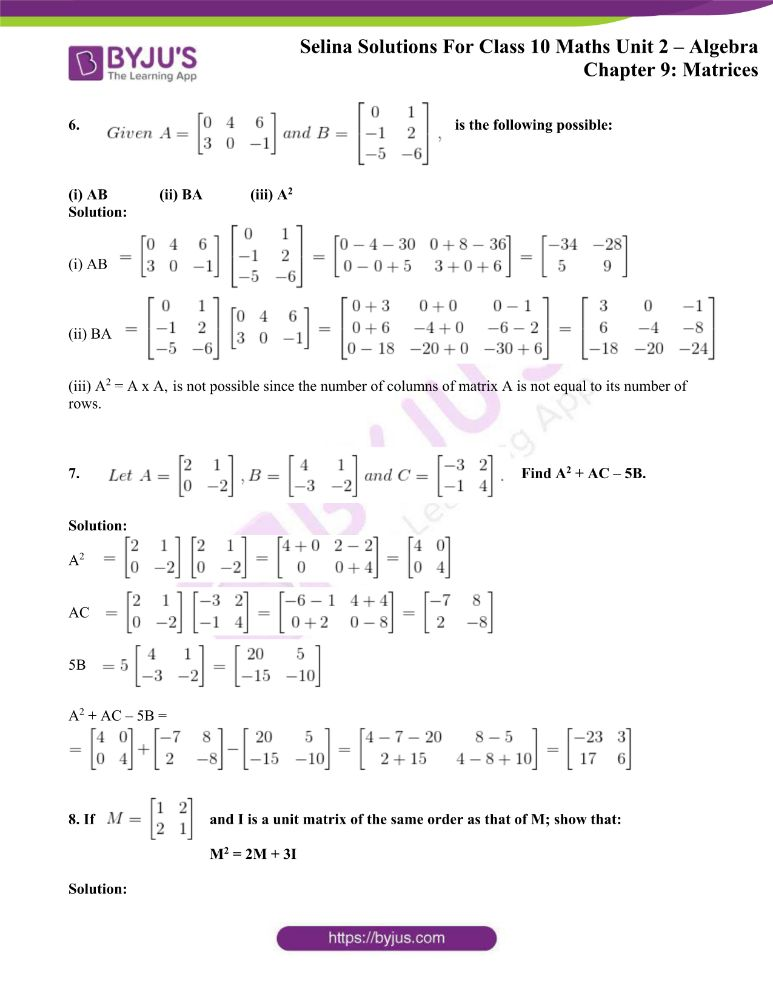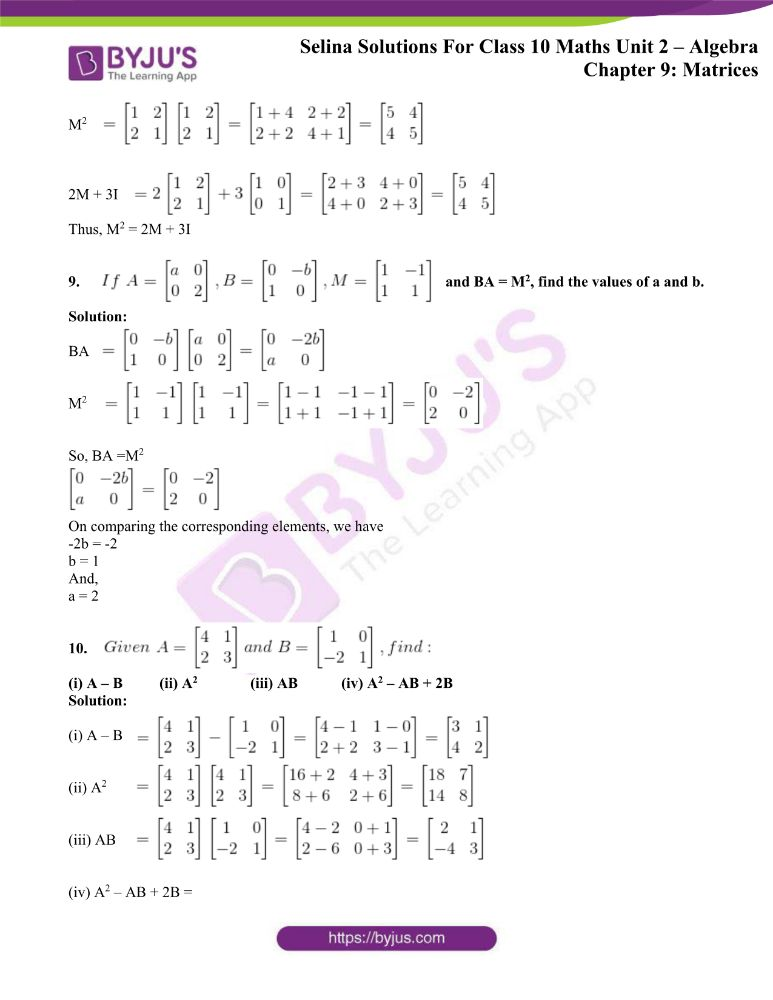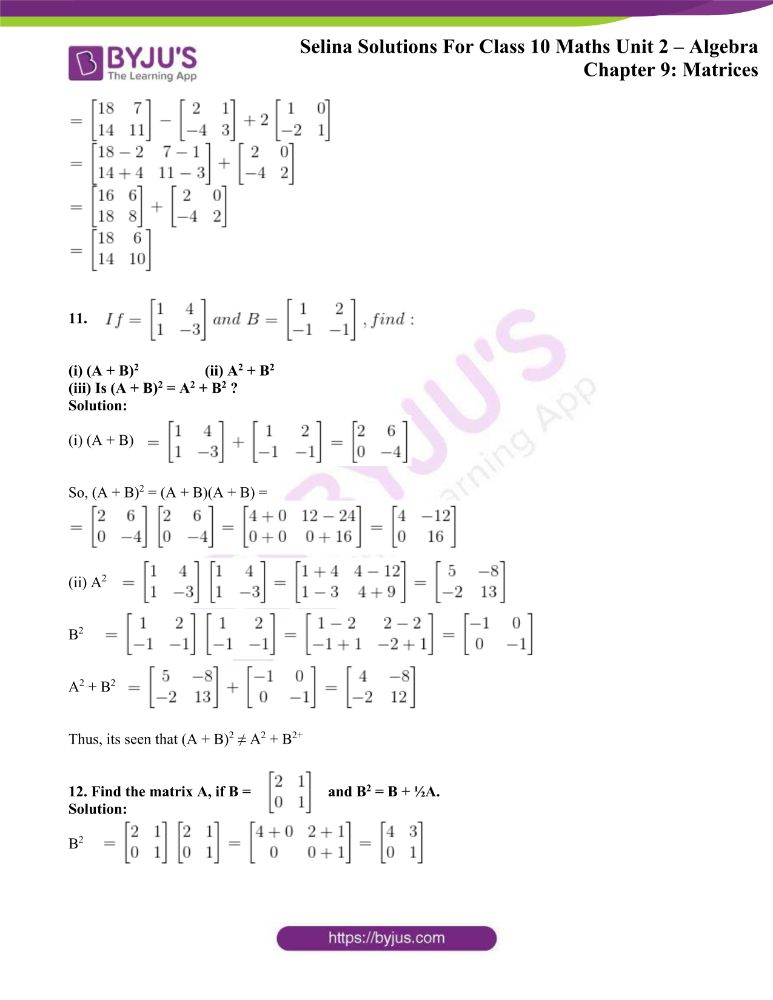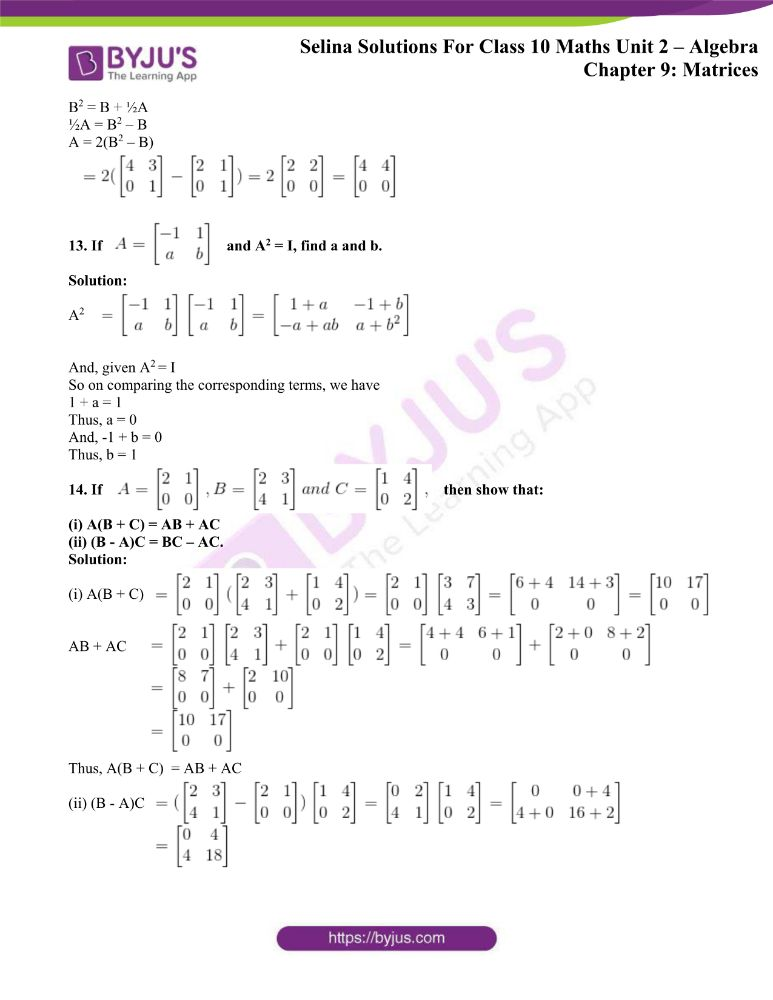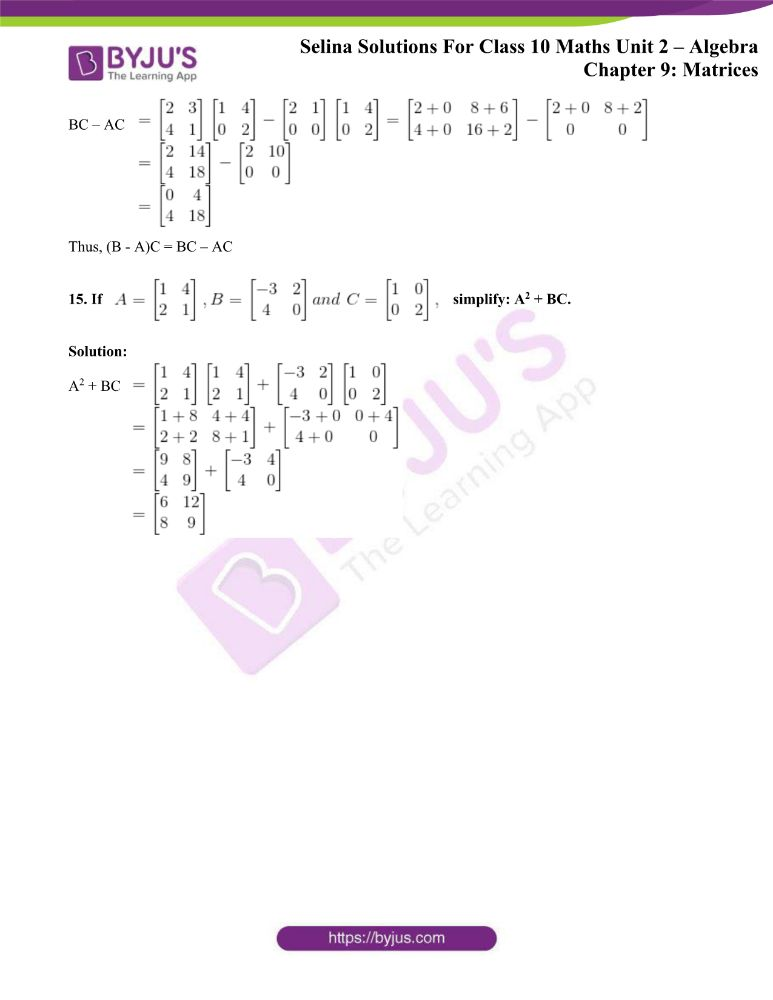### Access other exercises of Selina Solutions Concise Maths Class 10 Chapter 9 Matrices

Exercise 9(A) Solutions

Exercise 9(B) Solutions

Exercise 9(D) Solutions

### Access Selina Solutions Concise Maths Class 10 Chapter 9 Matrices Exercise 9(C)

1. Evaluate: if possible: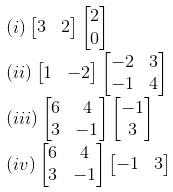If not possible, give reason.

Solution: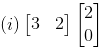= [6 + 0] =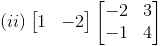= [-2+2 3-8] = [0 -5]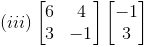=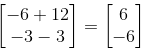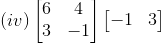The multiplication of these matrices is not possible as the rule for the number of columns in the first is not equal to the number of rows in the second matrix.

2. If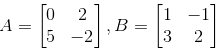and I is a unit matrix of order 2×2, find:

(i) AB (ii) BA (iii) AI

(iv) IB (v) A2 (iv) B2A

Solution:

(i) AB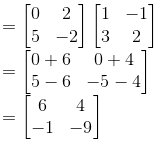(ii) BA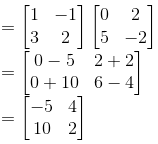(iii) AI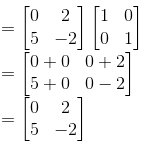(iv) IB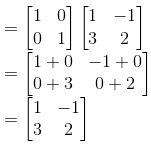(v) A2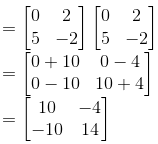(vi) B2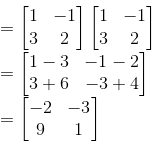B2A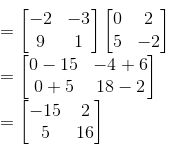3. If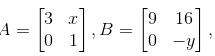find x and y when x and y when A2 = B.

Solution:

A2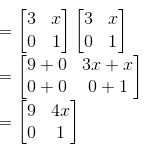A2 = B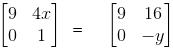On comparing corresponding elements, we have

4x = 16

x = 4

And,

1 = -y

y = -1

4. Find x and y, if: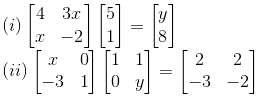Solution:

(i)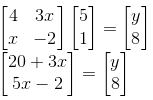On comparing the corresponding terms, we have

5x – 2 = 8

5x = 10

x = 2

And,

20 + 3x = y

20 + 3(2) = y

20 + 6 = y

y = 26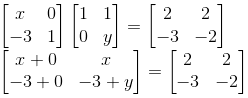(ii)

On comparing the corresponding terms, we have

x = 2

And,

-3 + y = -2

y = 3 – 2 = 1

5.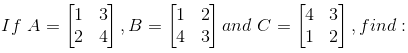(i) (AB) C (ii) A (BC)

Solution:

(i) (AB)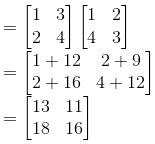(AB) C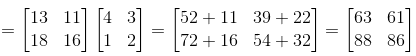(ii) BC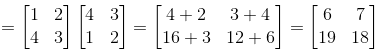A (BC)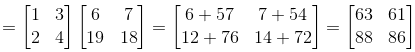Therefore, its seen that (AB) C = A (BC)

6.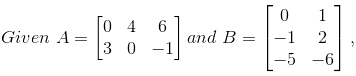is the following possible:

(i) AB (ii) BA (iii) A2

Solution:

(i) AB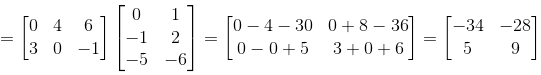(ii) BA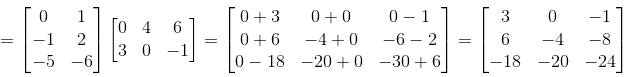(iii) A2 = A x A, is not possible since the number of columns of matrix A is not equal to its number of rows.

7.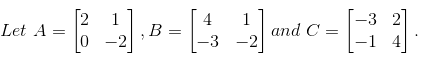Find A2 + AC – 5B.

Solution:

A2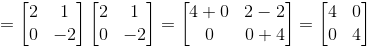AC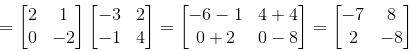5B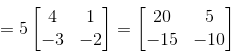A2 + AC – 5B =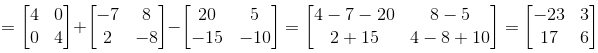8. If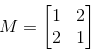and I is a unit matrix of the same order as that of M; show that:

M2 = 2M + 3I

Solution:

M2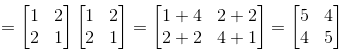2M + 3I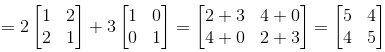Thus, M2 = 2M + 3I

9.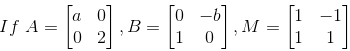and BA = M2, find the values of a and b.

Solution:

BA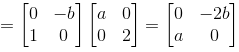M2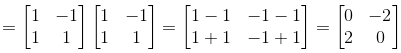So, BA =M2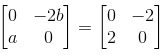On comparing the corresponding elements, we have

-2b = -2

b = 1

And,

a = 2

10.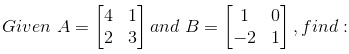(i) A – B (ii) A2 (iii) AB (iv) A2 – AB + 2B

Solution:

(i) A – B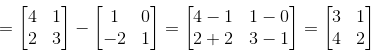(ii) A2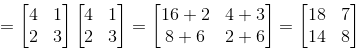(iii) AB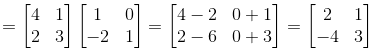(iv) A2 – AB + 2B =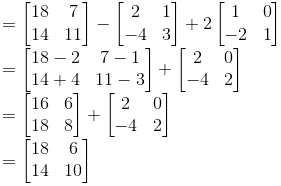11.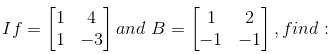(i) (A + B)2 (ii) A2 + B2

(iii) Is (A + B)2 = A2 + B2 ?

Solution:

(i) (A + B)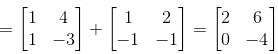So, (A + B)2 = (A + B)(A + B) =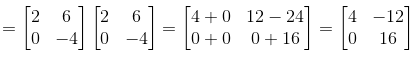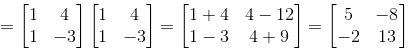(ii) A2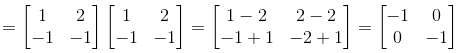B2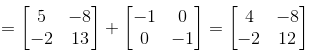A2 + B2

Thus, its seen that (A + B)2 ≠ A2 + B2+

12. Find the matrix A, if B =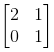and B2 = B + ½A.

Solution:

B2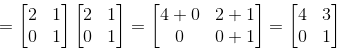B2 = B + ½A

½A = B2 – B

A = 2(B2 – B)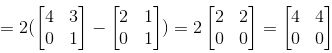13. If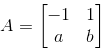and A2 = I, find a and b.

Solution: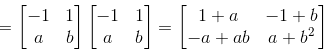A2

And, given A2 = I

So on comparing the corresponding terms, we have

1 + a = 1

Thus, a = 0

And, -1 + b = 0

Thus, b = 1

14. If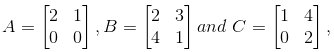then show that:

(i) A(B + C) = AB + AC

(ii) (B – A)C = BC – AC.

Solution:

(i) A(B + C)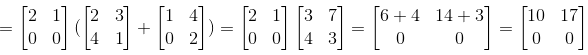AB + AC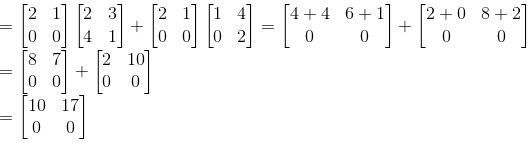Thus, A(B + C) = AB + AC

(ii) (B – A)C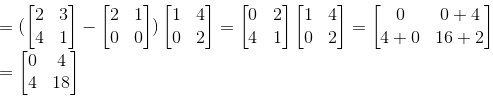BC – AC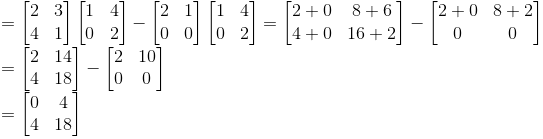Thus, (B – A)C = BC – AC

15. If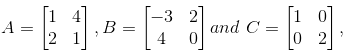simplify: A2 + BC.

Solution:

A2 + BC